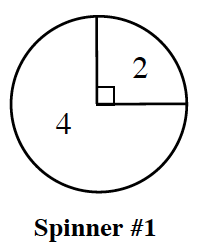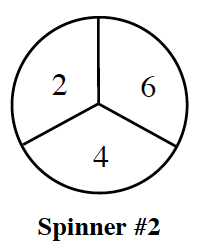### Home > CCG > Chapter 5 > Lesson 5.3.3 > Problem5-105

5-105.

The spinners at right are spun and the results are added.

1. Find $\text{P}(\text{sum is }4)$.

Draw an area model or tree diagram.

2. Find $\text{P}(\text{sum is }8)$.

Using the area model or tree diagram you made in part (a), circle all the places on your model where the sum is $8$.

$P(\text{sum is }8)=\frac{1}{12}+\frac{1}{4}=\frac{1}{3}$